##### Excel Dashboards & Reports For Dummies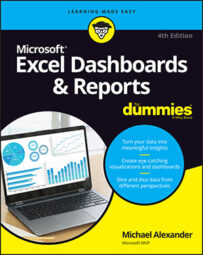The SUMPRODUCT function is actually listed under the math and trigonometry category of Excel functions. Because the primary purpose of SUMPRODUCT is to calculate the sum product, most people don't know you can actually use it to look up values. In fact, you can use this versatile function quite effectively in most data models.

## SUMPRODUCT basics

The SUMPRODUCT function is designed to multiply values from two or more ranges of data and then add the results together to return the sum of the products. Take a look at the following figure to see a typical scenario in which the SUMPRODUCT is useful.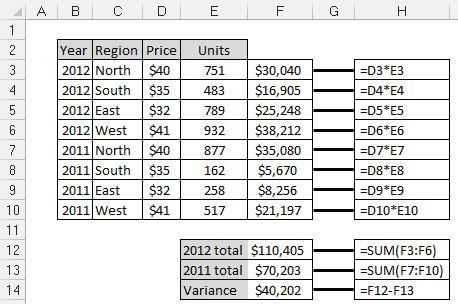SUMPRODUCT, getting the total sales involves multiplying pric" width="458"/>
Without the SUMPRODUCT, getting the total sales involves multiplying price by units and then summing the results.

You see a common analysis in which you need the total sales for the years 2011 and 2012. As you can see, to get the total sales for each year, you first have to multiply Price by the number of Units to get the total for each Region. Then you have to sum those results to get the total sales for each year.

With the SUMPRODUCT function, you can perform the two-step analysis with just one formula. The following figure shows the same analysis with SUMPRODUCT formulas. Rather than use 11 formulas, you can accomplish the same analysis with just 3!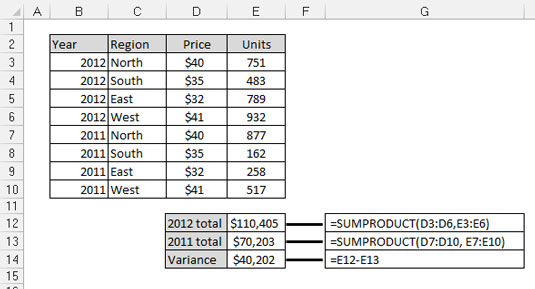SUMPRODUCT function allows you to perform the same analysis with just" width="535"/>
The SUMPRODUCT function allows you to perform the same analysis with just 3 formulas instead of 11.

The syntax of the SUMPRODUCT function is fairly simple:

`SUMPRODUCT(<i>Array1</i>, <i>Array2</i>, …)`

Array: Array represents a range of data. You can use anywhere from 2 to 255 arrays in a SUMPRODUCT formula. The arrays are multiplied together and then added. The only hard-and-fast rule you have to remember is that all arrays must have the same number of values. That is to say, you can't use the SUMPRODUCT if range X has 10 values and range Y has 11 values. Otherwise, you get the #VALUE! error.

## A twist on the SUMPRODUCT function

The interesting thing about the SUMPRODUCT function is that it can be used to filter out values. Take a look at the following figure: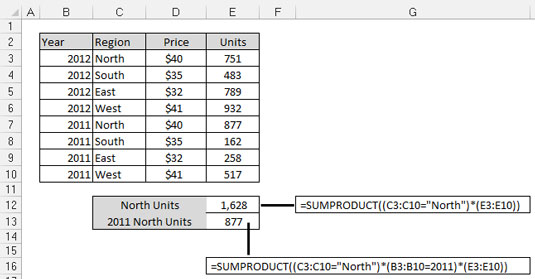SUMPRODUCT function can be used to filter data based on criteria." width="535"/>
The SUMPRODUCT function can be used to filter data based on criteria.

The formula in cell E12 is pulling the sum of total units for just the North region. Meanwhile, cell E13 is pulling the units logged for the North region in the year 2011.

To understand how this works, take a look at the formula in cell E12. That formula reads SUMPRODUCT((C3:C10="North")*(E3:E10)).

In Excel, TRUE evaluates to 1 and FALSE evaluates to 0. Every value in column C that equals North evaluates to TRUE or 1. Where the value is not North, it evaluates to FALSE or 0.The part of the formula that reads (C3:C10="North") enumerates through each value in the range C3:C10, assigning a 1 or 0 to each value. Then internally, the SUMPRODUCT formula translates to

`(1*E3)+(0*E4)+(0*E5)+(0*E6)+(1*E7)+(0*E8)+(0*E9)+(0*E10).`

This gives you the answer of 1628 because

`(1*751)+(0*483)+(0*789)+(0*932)+(1*877)+(0*162)+(0*258)+(0*517)`

equals 1628.

## Applying SUMPRODUCT formulas in a data model

As always in Excel, you don't have to hard-code the criteria in your formulas. Rather than explicitly use "North" in the SUMPRODUCT formula, you could reference a cell that contains the filter value. You can imagine that cell A3 contains the word North, in which case you can use (C3:C10=A3) instead of (C3:C10="North"). This way, you can dynamically change your filter criteria, and your formula keeps up.

The following figure demonstrates how you can use this concept to pull data into a staging table based on multiple criteria. Note that each of the SUMPRODUCT formulas shown here references cells B3 and C3 to filter on Account and Product Line. Again, you can add data validation drop-down lists to cells B3 and C3, allowing you to easily change criteria.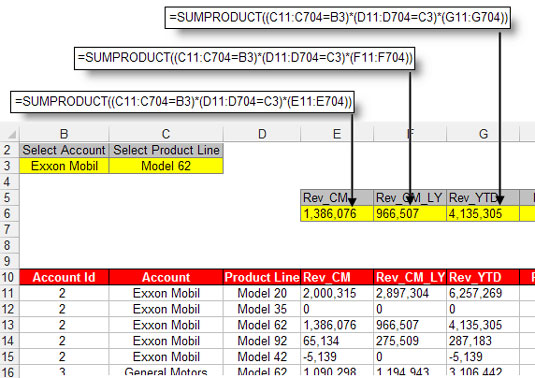SUMPRODUCT function can be used to pull summarized numbers from the d" width="535"/>
The SUMPRODUCT function can be used to pull summarized numbers from the data layer into staging tables.